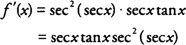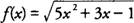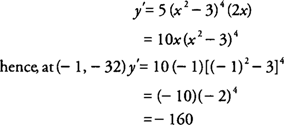## Chain Rule

The chain rule provides us a technique for finding the derivative of composite functions, with the number of functions that make up the composition determining how many differentiation steps are necessary. For example, if a composite function f( x) is defined asNote that because two functions, g and h, make up the composite function f, you have to consider the derivatives g′ and h′ in differentiating f( x).

If a composite function r( x) is defined asHere, three functions— m, n, and p—make up the composition function r; hence, you have to consider the derivatives m′, n′, and p′ in differentiating r( x). A technique that is sometimes suggested for differentiating composite functions is to work from the “outside to the inside” functions to establish a sequence for each of the derivatives that must be taken.

Example 1: Find f′( x) if f( x) = (3x 2 + 5x − 2) 8.Example 2: Find f′( x) if f( x) = tan (sec x).Example 3: Findif y = sin 3 (3 x − 1).Example 4: Find f′(2) ifExample 5: Find the slope of the tangent line to a curve y = ( x 2 − 3) 5 at the point (−1, −32).

Because the slope of the tangent line to a curve is the derivative, you find thatwhich represents the slope of the tangent line at the point (−1,−32).